tclusteda

tclusteda computes tclust for a series of values of the trimming factor

Syntax

• out=tclusteda(Y,k,alpha,restrfactor)example
• out=tclusteda(Y,k,alpha,restrfactor,Name,Value)example
• [out,varargout]=tclusteda(___)example

Description

tclusteda performs tclust for a series of values of the trimming factor alpha given k (number of groups) and given c (restriction factor). In order to increase the speed of the computations, parfor is used.

out =tclusteda(Y, k, alpha, restrfactor) Monitoring using geyser data (all default options).

out =tclusteda(Y, k, alpha, restrfactor, Name, Value) Monitoring using geyser data with alpha and c specified.

[out, varargout] =tclusteda(___) Monitoring using geyser data with option plots supplied as structure.

Examples

expand allMonitoring using geyser data (all default options).

close all
% alpha and restriction factor are not specified therefore for alpha
% vector [0.10 0.05 0] is used while for the restriction factor, value c=12
% is used
k=3;
[out]=tclusteda(Y,k);
Warning: You have not specified alpha: it is set to [0.10 0.05 0] by default
Warning: You have not specified restrfactor: it is set to 12 by default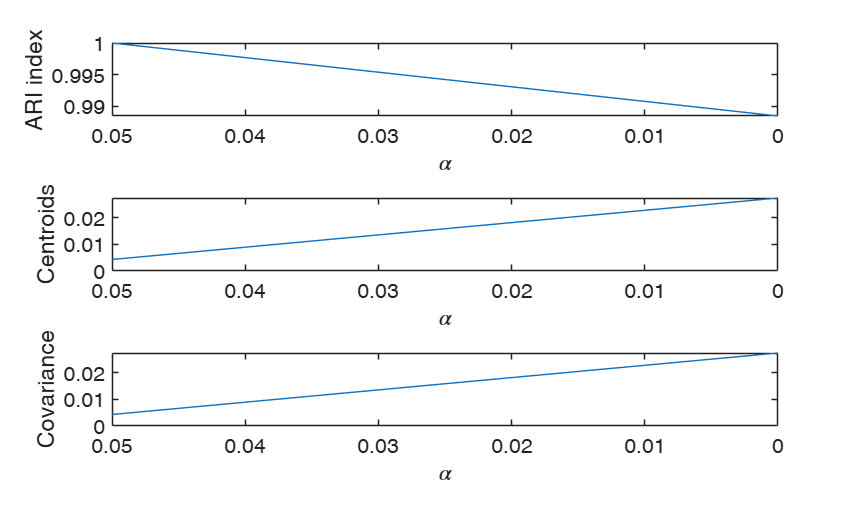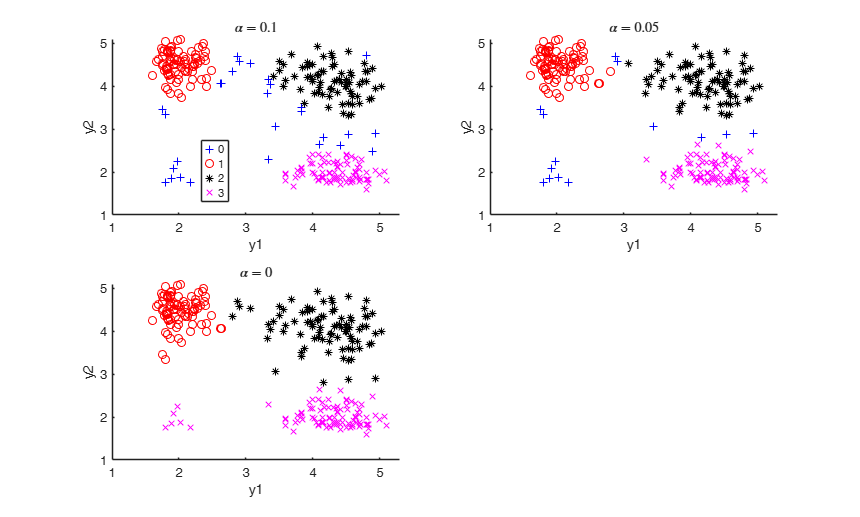Monitoring using geyser data with alpha and c specified.

close all
% alphavec= vector which contains the trimming levels to consider
alphavec=0.10:-0.01:0;
% c = restriction factor to use
c=100;
% k= number of groups
k=3;
[out]=tclusteda(Y,k,alphavec,c);Monitoring using geyser data with option plots supplied as structure.

close all
% alphavec= vector which contains the trimming levels to consider
% in this case 31 values of alpha are considered
alphavec=0.30:-0.01:0;
% c = restriction factor to use
c=100;
% k= number of groups
k=3;
% The monitoring plot of allocation will shows just these four values of
% alpha
plots=struct;
plots.alphasel=[0.2 0.10 0.05 0.01];
[out]=tclusteda(Y,k,alphavec,c,'plots',plots);
ClaLik with untrimmed units selected using crisp criterion
100%[===================================================]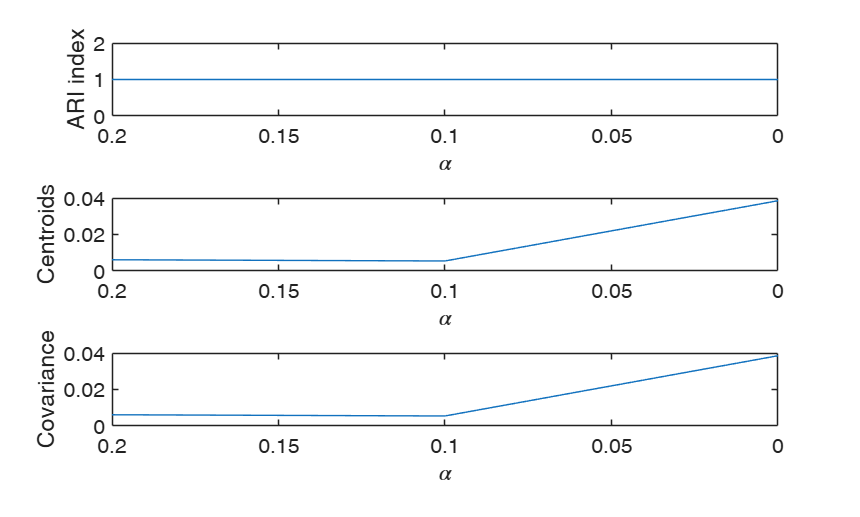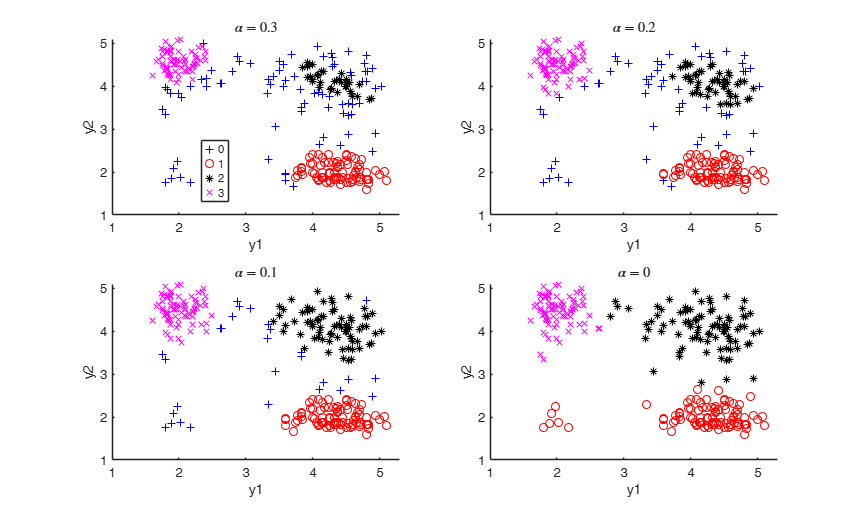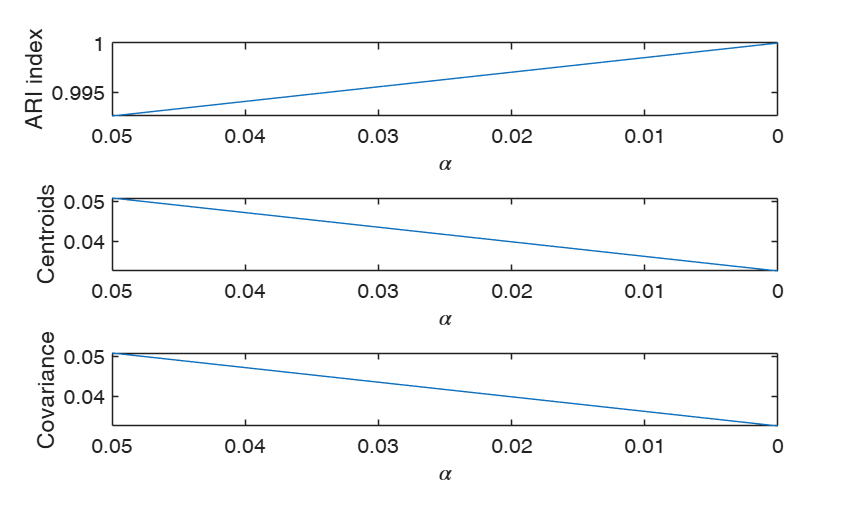Related Examples

expand allMonitoring geyser data with option UnitsSameGroup.

close all
% alphavec= vector which contains the trimming levels to consider
alphavec=0.30:-0.10:0;
% c = restriction factor to use
c=100;
% k= number of groups
k=3;
% Make sure that group containing unit 10 is in a group which is labelled
% group 1 and group containing unit 12 is in group which is labelled group 2
UnitsSameGroup=[10 12];
% Mixture model is used
mixt=2;
[out]=tclusteda(Y,k,alphavec,1000,'mixt',2,'UnitsSameGroup',UnitsSameGroup);
MixLik with untrimmed units selected using h largest likelihood contributions
100%[===================================================]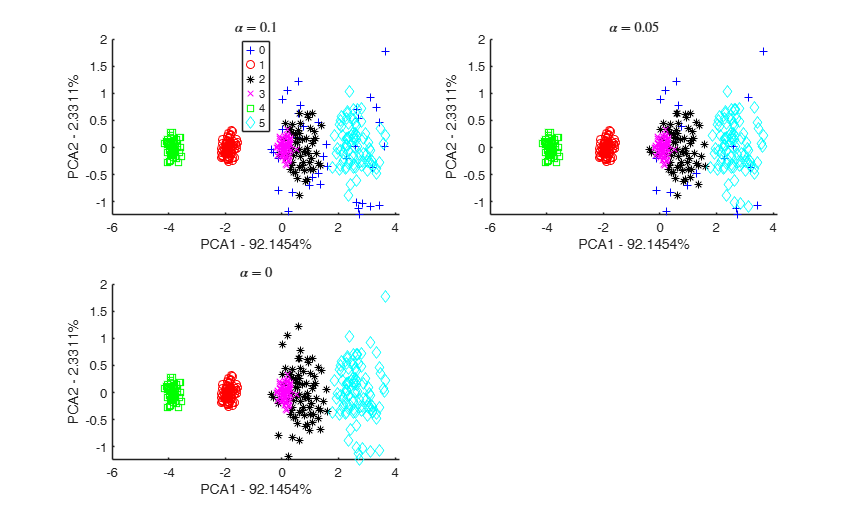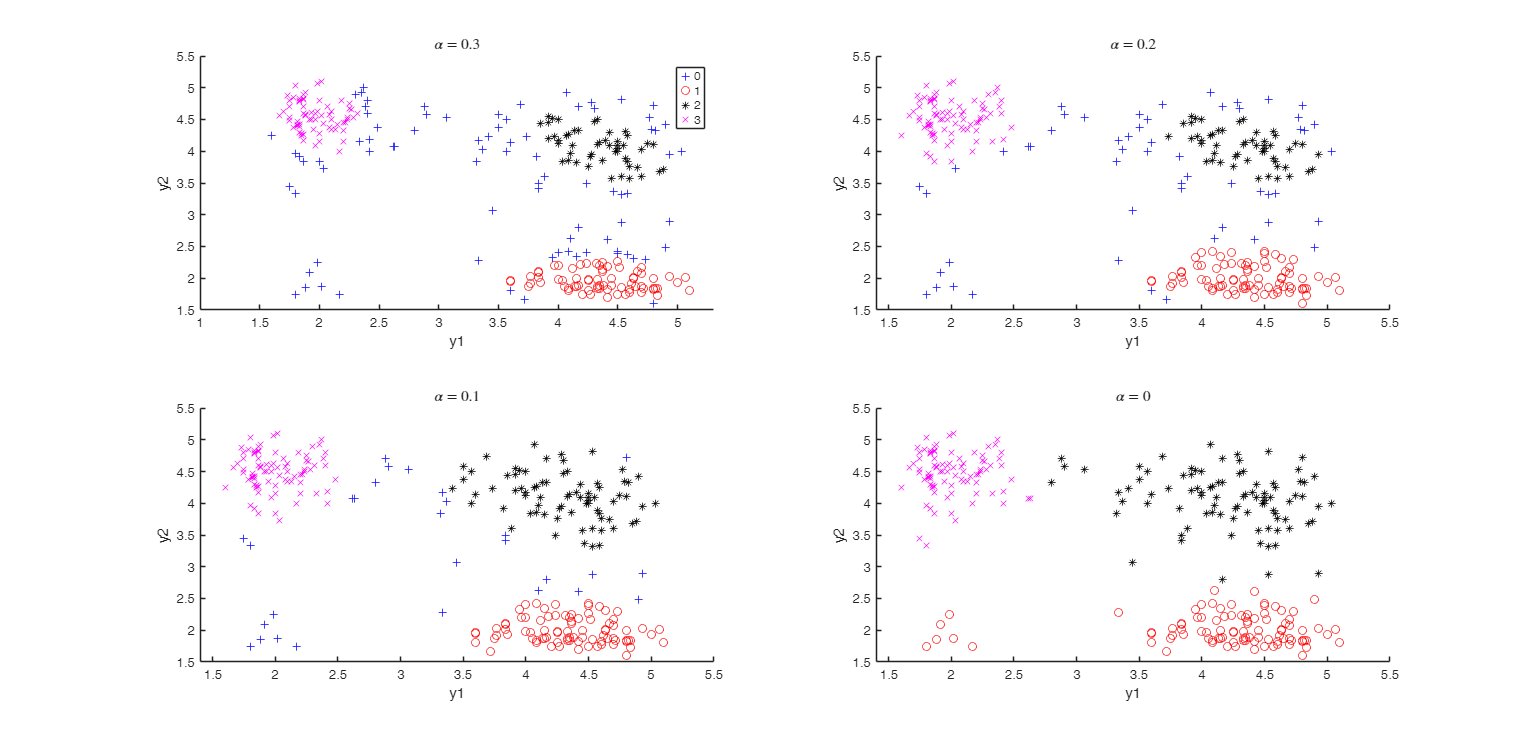tclusteda with M5 data.

close all
% alphavec= vector which contains the trimming levels to consider
alphavec=0.10:-0.02:0;
out=tclusteda(Y(:,1:2),3,alphavec,1000,'nsamp',1000,'plots',1);
ClaLik with untrimmed units selected using crisp criterion
100%[===================================================]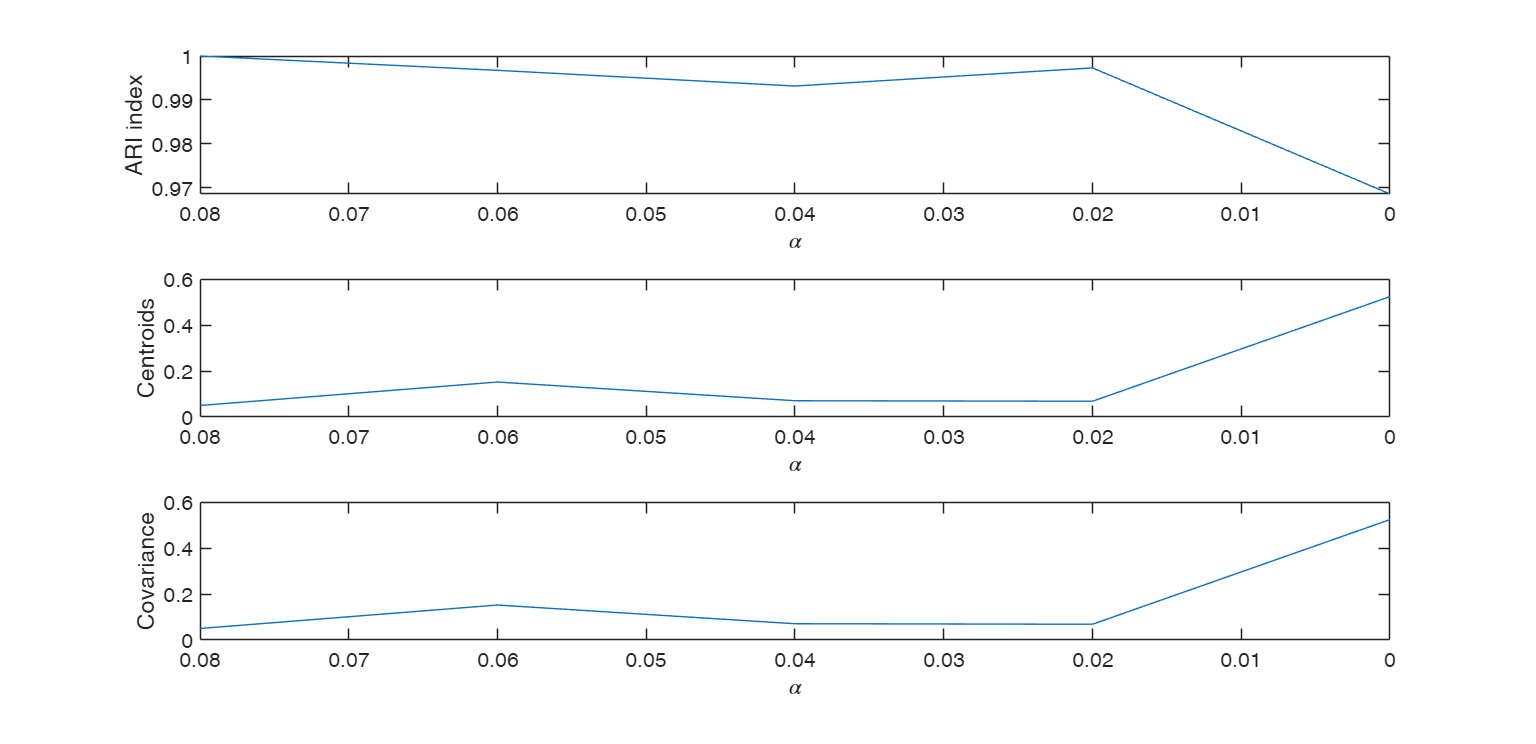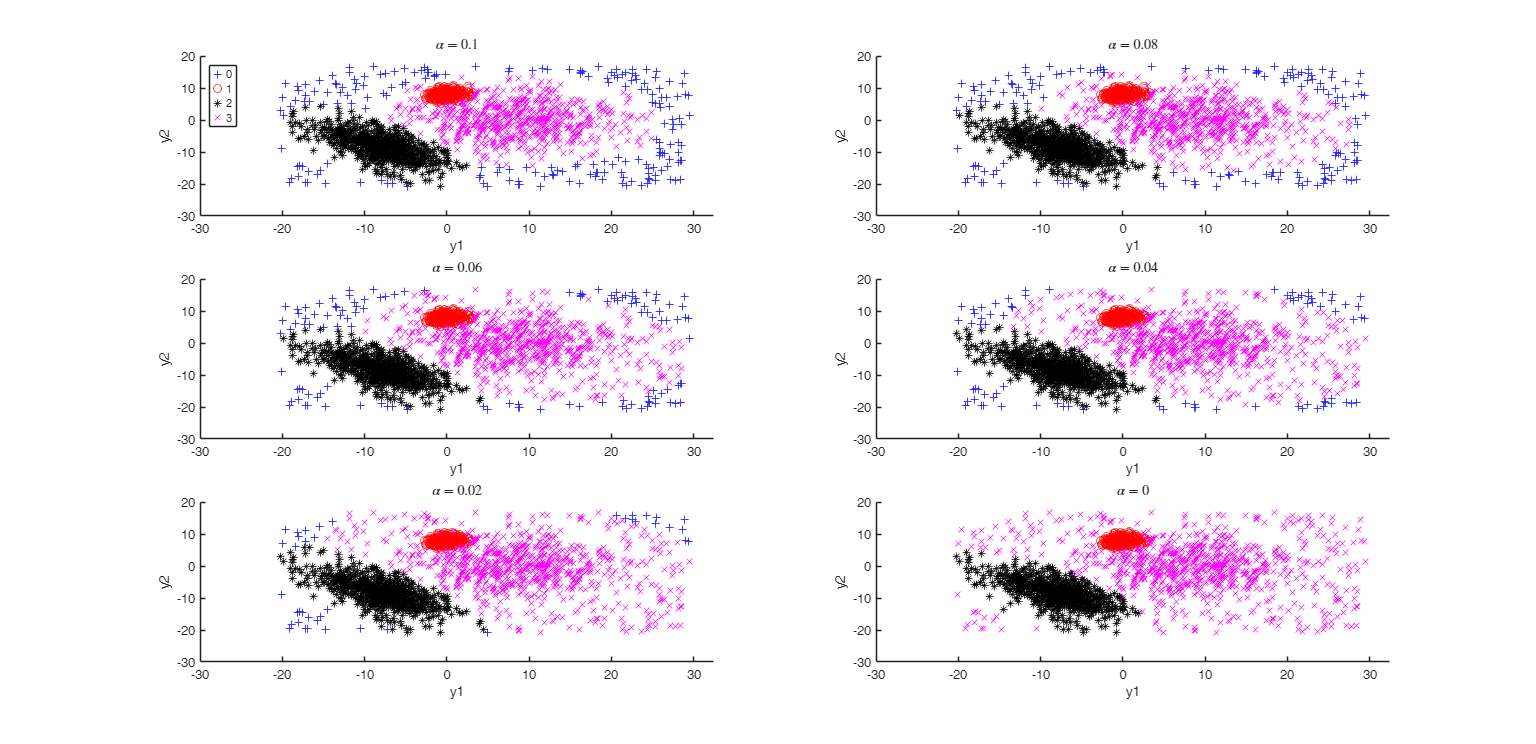Structured noise data ex1.

close all
alphavec=0.20:-0.01:0;
out=tclusteda(Y,2,alphavec,100,'plots',1);

Structured noise data ex2.

close all
alphavec=0.20:-0.01:0;
% just show the monitoring plot
plots=struct;
plots.name = {'monitor'};
out=tclusteda(Y,2,alphavec,100,'plots',plots);

mixture100 data.

close all
alphavec=0.20:-0.01:0;
% just show the allocation plot
plots=struct;
plots.name = {'gscatter'};
out=tclusteda(Y,2,alphavec,100,'plots',plots);tclusteda using simulated data.

5 groups and 5 variables

rng(100,'twister')
n1=100;
n2=80;
n3=50;
n4=80;
n5=70;
v=5;
Y1=randn(n1,v)+5;
Y2=randn(n2,v)+3;
Y3=rand(n3,v)-2;
Y4=rand(n4,v)+2;
Y5=rand(n5,v);
group=ones(n1+n2+n3+n4+n5,1);
group(n1+1:n1+n2)=2;
group(n1+n2+1:n1+n2+n3)=3;
group(n1+n2+n3+1:n1+n2+n3+n4)=4;
group(n1+n2+n3+n4+1:n1+n2+n3+n4+n5)=5;
close all
Y=[Y1;Y2;Y3;Y4;Y5];
n=size(Y,1);
% Set number of groups
k=5;
% Example of the subsets precalculated
nsamp=2000;
nsampscalar=nsamp;
nsamp=subsets(nsamp,n,(v+1)*k);
% Random numbers to compute proportions computed once and for all
RandNumbForNini=rand(k,nsampscalar);
% The allocation is shown on the space of the first two principal
% components
out=tclusteda(Y,k,[],6,'plots',1,'RandNumbForNini',RandNumbForNini,'nsamp',nsamp);
Warning: You have not specified alpha: it is set to [0.10 0.05 0] by default
ClaLik with untrimmed units selected using crisp criterion
100%[===================================================]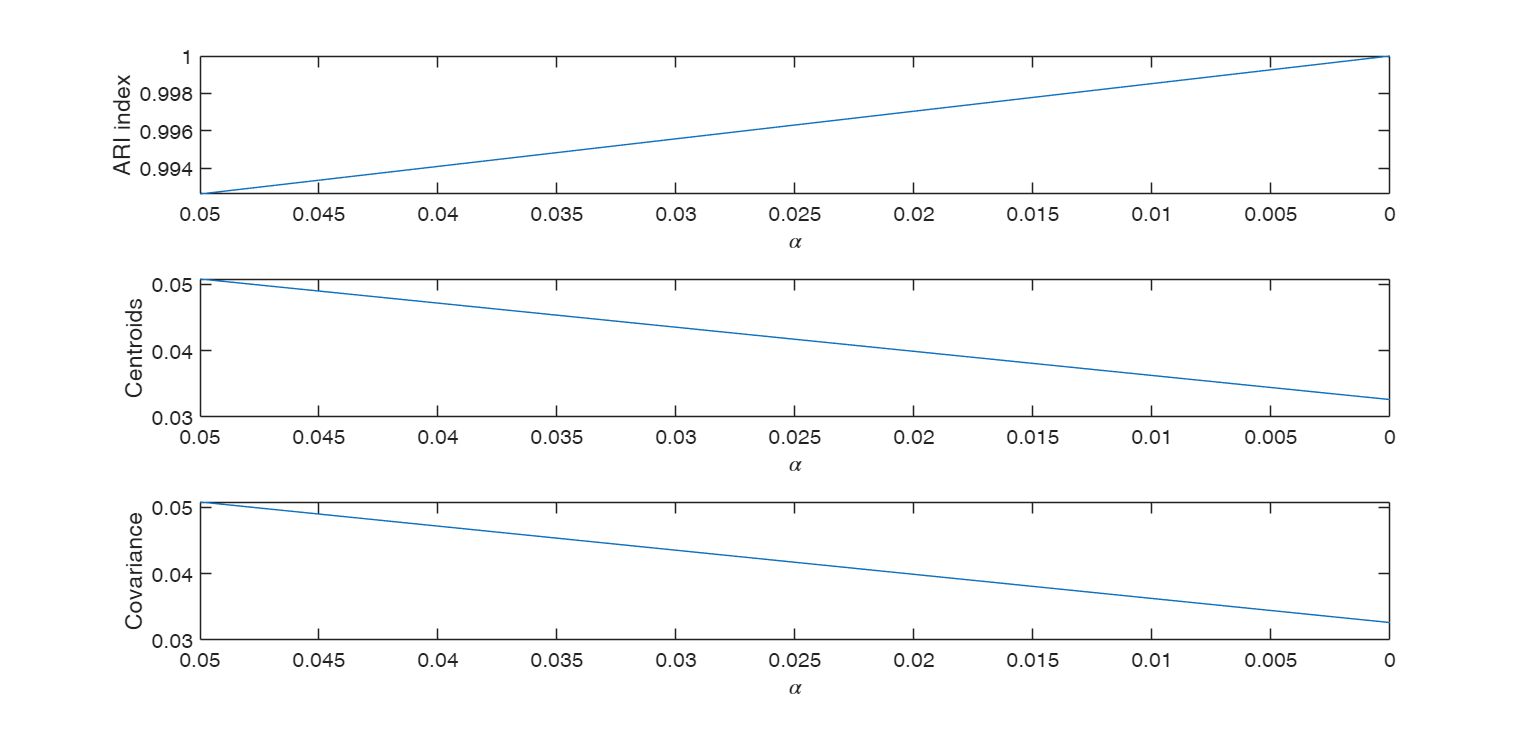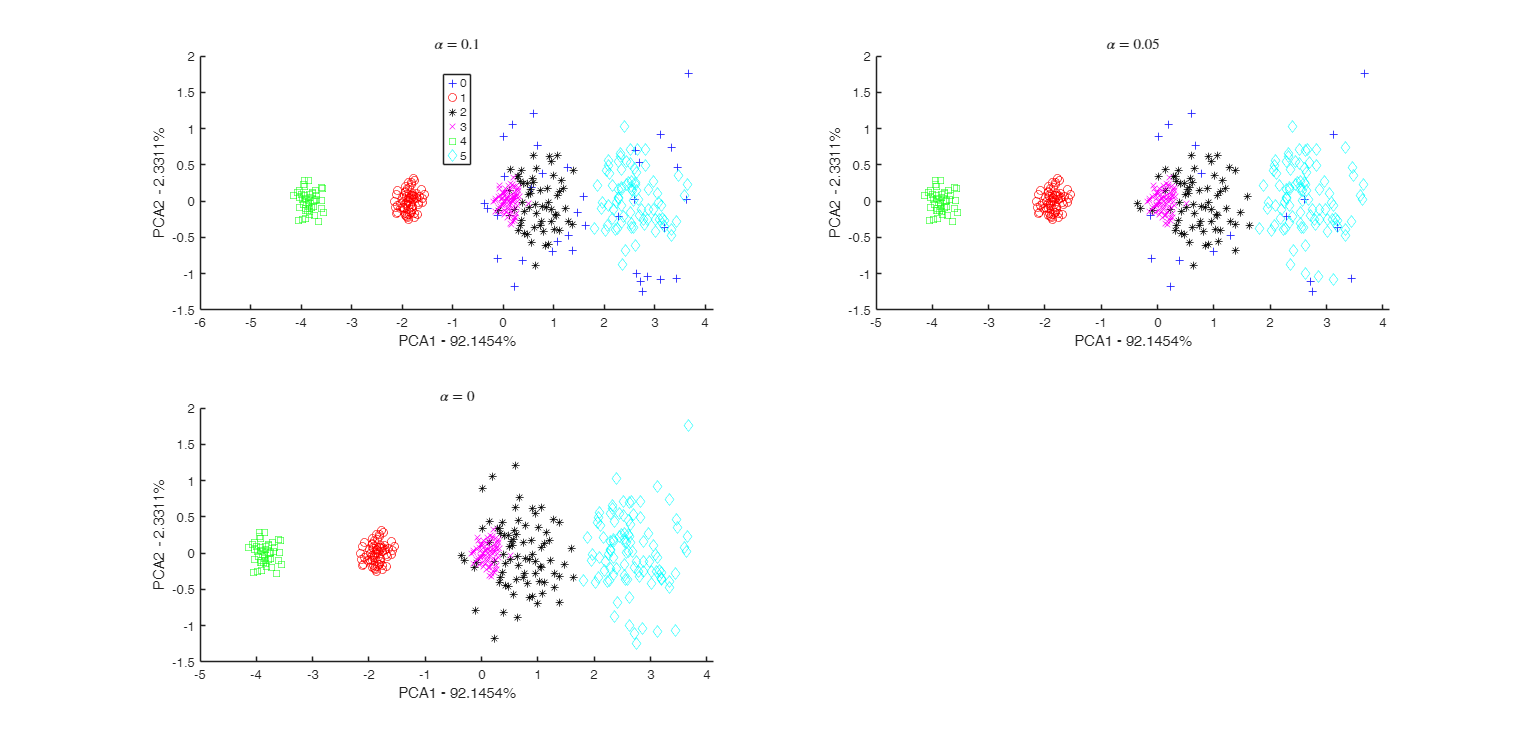tclusteda using determinant constraint.

Search for spherical clusters.

% 5 groups and 5 variables
rng(100,'twister')
n1=100;
n2=80;
n3=50;
n4=80;
n5=70;
v=5;
Y1=randn(n1,v)+5;
Y2=randn(n2,v)+3;
Y3=rand(n3,v)-2;
Y4=rand(n4,v)+2;
Y5=rand(n5,v);
group=ones(n1+n2+n3+n4+n5,1);
group(n1+1:n1+n2)=2;
group(n1+n2+1:n1+n2+n3)=3;
group(n1+n2+n3+1:n1+n2+n3+n4)=4;
group(n1+n2+n3+n4+1:n1+n2+n3+n4+n5)=5;
close all
Y=[Y1;Y2;Y3;Y4;Y5];
n=size(Y,1);
% Set number of groups
k=5;
cshape=1
out=tclusteda(Y,k,[],1000,'plots',1,'restrtype','deter','cshape',cshape);

An example of use of plots as a structure with field ylimy.

Y=swiss_banknotes{:,:};
[n,v]=size(Y);
alphavec=0.15:-0.01:0;
% alphavec=0.12:-0.005:0;
% c = restriction factor to use
c=100;
% k= number of groups
k=2;
% restriction on the determinants is imposed
restrtype='deter';
% Specify lower and upper limits for the monitoring plot
plots=struct;
% ylimits for monitoring of ARI index
ylimARI=[0.95 1];
% ylimits for change in centroids
ylimCENT=[0 0.02];
% ylimits for change in cov matrices
ylimCOV=[0 0.01];
ylimy=[ylimARI;ylimCENT;ylimCOV];
plots.ylimy=ylimy;
[outDet]=tclusteda(Y,k,alphavec,c,'restrtype',restrtype,'plots',plots,'nsamp',10000);

Input Arguments

Y — Input data. Matrix.

Data matrix containing n observations on v variables.

Rows of Y represent observations, and columns represent variables.

Missing values (NaN's) and infinite values (Inf's) are allowed, since observations (rows) with missing or infinite values will automatically be excluded from the computations.

Data Types: single| double

k — Number of groups. Scalar.

Scalar which specifies the number of groups.

Data Types: single| double

alpha — trimming level to monitor. Vector.

Vector which specifies the values of trimming levels which have to be considered.

alpha is a vector which contains decreasing elements which lie in the interval 0 and 0.5.

For example if alpha=[0.1 0.05 0] tclusteda considers these 3 values of trimming level.

If alpha=0 tclusteda reduces to traditional model based or mixture clustering (mclust): see Matlab function gmdistribution. The default for alpha is vector [0.1 0.05 0]. The sequence is forced to be monotonically decreasing.

Data Types: single| double

restrfactor — Restriction factor. Scalar.

Positive scalar which constrains the allowed differences among group scatters. Larger values imply larger differences of group scatters. On the other hand a value of 1 specifies the strongest restriction forcing all eigenvalues/determinants to be equal and so the method looks for similarly scattered (respectively spherical) clusters. The default is to apply restrfactor to eigenvalues. In order to apply restrfactor to determinants it is is necessary to use optional input argument cshape.

Data Types: single| double

Name-Value Pair Arguments

Specify optional comma-separated pairs of Name,Value arguments. Name is the argument name and Value is the corresponding value. Name must appear inside single quotes (' '). You can specify several name and value pair arguments in any order as Name1,Value1,...,NameN,ValueN.

Example: 'nsamp',1000 , 'refsteps',10 , 'reftol',1e-05 , 'equalweights',true , 'mixt',1 , 'plots', 1 , 'msg',1 , 'nocheck',1 , 'startv1',1 , 'RandNumbForNini','' , 'restrtype','deter' , 'cshape',10 , 'UnitsSameGroup',[20 34] , 'numpool',4 , 'cleanpool',true

nsamp —Number of subsamples to extract.scalar | matrix.

If nsamp is a scalar it contains the number of subsamples which will be extracted. If nsamp=0 all subsets will be extracted.

Remark - if the number of all possible subset is <300 the default is to extract all subsets, otherwise just 300 If nsamp is a matrix it contains in the rows the indexes of the subsets which have to be extracted. nsamp in this case can be conveniently generated by function subsets. nsamp can have k columns or k*(v+1) columns. If nsamp has k columns the k initial centroids each iteration i are given by X(nsamp(i,:),:) and the covariance matrices are equal to the identity.

If nsamp has k*(v+1) columns the initial centroids and covariance matrices in iteration i are computed as follows:

X1=X(nsamp(i,:),:);

mean(X1(1:v+1,:)) contains the initial centroid for group 1;

cov(X1(1:v+1,:)) contains the initial cov matrix for group 1;

mean(X1(v+2:2*v+2,:)) contains the initial centroid for group 2;

cov((v+2:2*v+2,:)) contains the initial cov matrix for group 2;

...;

mean(X1((k-1)*v+1:k*(v+1))) contains the initial centroids for group k;

cov(X1((k-1)*v+1:k*(v+1))) contains the initial cov matrix for group k.

REMARK - if nsamp is not a scalar option option below startv1 is ignored. More precisely, if nsamp has k columns startv1=0 elseif nsamp has k*(v+1) columns option startv1 =1.

Example: 'nsamp',1000

Data Types: double

refsteps —Number of refining iterations.scalar.

Number of refining iterations in each subsample. Default is 15.

refsteps = 0 means "raw-subsampling" without iterations.

Example: 'refsteps',10

Data Types: single | double

reftol —Tolerance for the refining steps.scalar.

The default value is 1e-14;

Example: 'reftol',1e-05

Data Types: single | double

equalweights —Cluster weights in the concentration and assignment steps.logical.

A logical value specifying whether cluster weights shall be considered in the concentration, assignment steps and computation of the likelihood.

if equalweights = true we are (ideally) assuming equally sized groups by maximizing:

$\sum_{j=1}^k \sum_{ x_i \in group_j } \log f(x_i; m_j , S_j)$

else if equalweights = false (default) we allow for different group weights by maximizing:

$\sum_{j=1}^k \sum_{ x_i \in group_j } \log \left[ \frac{n_j}{n} f(x_i; m_j , S_j) \right]=$ $= \sum_{j=1}^k n_j \log n_j/n + \sum_{j=1}^k \sum_{ x_i \in group_j} \log f(x_i; m_j , S_j) .$ Remark: $\sum_{j=1}^k n_j \log n_j/n$ is the so called entropy term

Example: 'equalweights',true

Data Types: Logical

mixt —Mixture modelling or crisp assignment.scalar.

Option mixt specifies whether mixture modelling or crisp assignment approach to model based clustering must be used.

In the case of mixture modelling parameter mixt also controls which is the criterion to find the untrimmed units in each step of the maximization.

If mixt >=1 mixture modelling is assumed else crisp assignment. The default value is mixt=0 (i.e. crisp assignment).

In mixture modelling the likelihood is given by:

$\prod_{i=1}^n \sum_{j=1}^k \pi_j \phi (y_i; \; \theta_j),$

while in crisp assignment the likelihood is given by:

$\prod_{j=1}^k \prod _{i\in R_j} \phi (y_i; \; \theta_j),$

where $R_j$ contains the indexes of the observations which are assigned to group $j$.

Remark - if mixt>=1 previous parameter equalweights is automatically set to 1.

Parameter mixt also controls the criterion to select the units to trim, if mixt = 2 the h units are those which give the largest contribution to the likelihood that is the h largest values of:

$\sum_{j=1}^k \pi_j \phi (y_i; \; \theta_j) \qquad i=1, 2, ..., n,$

else if mixt=1 the criterion to select the h units is exactly the same as the one which is used in crisp assignment. That is: the n units are allocated to a cluster according to criterion:

$\max_{j=1, \ldots, k} \hat \pi'_j \phi (y_i; \; \hat \theta_j)$

and then these n numbers are ordered and the units associated with the largest h numbers are untrimmed.

Example: 'mixt',1

Data Types: single | double

plots —Plot on the screen.scalar structure.

Case 1: plots option used as scalar.

- If plots=0, plots are not generated.

- If plots=1 (default), two plots are shown on the screen.

The first plot ("monitor plot") shows three panels monitoring between two consecutive values of alpha the change in classification using ARI index (top panel), the change in centroids using squared euclidean distances (central panel), the change in covariance matrices using squared euclidean distance (bottom panel).

The second plot ("gscatter plot") shows a series of subplots which monitor the classification for each value of alpha. In order to make sure that consistent labels are used for the groups, between two consecutive values of alpha, we assign label r to a group if this group shows the smallest distance with group r for the previous value of alpha. The type of plot which is used to monitor the stability of the classification depends on the value of v.

* for v=1, we use histograms of the univariate data (function histFS is called).

* for v=2, we use the scatter plot of the two variables (function gscatter is called).

* for v>2, we use the scatter plot of the first two principal components (function gscatter is called and we show on the axes titles the percentage of variance explained by the first two principal components).

Case 2: plots option used as struct.

If plots is a structure it may contain the following fields:

Value Description
name

cell array of strings which enables to specify which plot to display. plots.name = {'gscatter'} produces a figure with a series of subplots which show the classification for each value of alpha. plots.name = {'monitor'} shows a figure with 3 panels which monitor between two consecutive values of alpha the change in classification using ARI index (top panel), the change in centroids using squared euclidean distances (central panel), the change in covariance matrices using squared euclidean distance (bottom panel). If this field is not specified plots.name={'gscatter' 'monitor'} and both figures will be shown.

alphasel

numeric vector which speciies for which values of alpha it is possible to see the classification.

For example if plots.alphasel =[ 0.05 0.02], the classification will be shown just for alpha=0.05 and alpha=0.02; If this field is not specified plots.alphasel=alpha and therefore the classification is shown for each value of alpha.

ylimy

2D array of size 3-by 2 which specifies the lower and upper limits for the monitoring plots. The first row refers the ARI index (top panel), the second row refers to the the change in centroids using squared euclidean distances (central panel), the third row is associated with the change in covariance matrices using squared euclidean distance (bottom panel).

Example: 'plots', 1

Data Types: single | double | struct

msg —Level of output to display.scalar.

Scalar which controls whether to display or not messages on the screen.

If msg=0 nothing is displayed on the screen.

If msg=1 (default) messages are displayed on the screen about estimated time to compute the estimator or the number of subsets in which there was no convergence.

If msg=2 detailed messages are displayed. For example the information at iteration level.

Example: 'msg',1

Data Types: single | double

nocheck —Check input arguments.scalar.

If nocheck is equal to 1 no check is performed on matrix Y.

As default nocheck=0.

Example: 'nocheck',1

Data Types: single | double

startv1 —How to initialize centroids and covariance matrices.scalar.

If startv1 is 1 then initial centroids and covariance matrices are based on (v+1) observations randomly chosen, else each centroid is initialized taking a random row of input data matrix and covariance matrices are initialized with identity matrices. The default value of startv1 is 1.

Remark 1 - in order to start with a routine which is in the required parameter space, eigenvalue restrictions are immediately applied.

Remark 2 - option startv1 is used just if nsamp is a scalar (see for more details the help associated with nsamp).

Example: 'startv1',1

Data Types: single | double

RandNumbForNini —Pre-extracted random numbers to initialize proportions.matrix.

Matrix with size k-by-size(nsamp,1) containing the random numbers which are used to initialize the proportions of the groups. This option is effective just if nsamp is a matrix which contains pre-extracted subsamples. The purpose of this option is to enable to user to replicate the results in case routine tclust is called using a parfor instruction (as it happens for example in routine tclustIC, where tclust is called through a parfor for different values of the restriction factor).

The default value of RandNumbForNini is empty that is random numbers from uniform are used.

Example: 'RandNumbForNini',''

Data Types: single | double

restrtype —type of restriction.character.

The type of restriction to be applied on the cluster scatter matrices. Valid values are 'eigen' (default), or 'deter'.

eigen implies restriction on the eigenvalues while deter implies restriction on the determinants.

Example: 'restrtype','deter'

Data Types: char

cshape —constraint to apply to each of the shape matrices.scalar greater | equal than 1.

This options only works is 'restrtype' is 'deter'.

When restrtype is deter the default value of the "shape" constraint (as defined below) applied to each group is fixed to $c_{shape}=10^{10}$, to ensure the procedure is (virtually) affine equivariant. In other words, the decomposition or the $j$-th scatter matrix $\Sigma_j$ is

$\Sigma_j=\lambda_j^{1/v} \Omega_j \Gamma_j \Omega_j'$

where $\Omega_j$ is an orthogonal matrix of eigenvectors, $\Gamma_j$ is a diagonal matrix with $|\Gamma_j|=1$ and with elements $\{\gamma_{j1},...,\gamma_{jv}\}$ in its diagonal (proportional to the eigenvalues of the $\Sigma_j$ matrix) and $|\Sigma_j|=\lambda_j$. The $\Gamma_j$ matrices are commonly known as "shape" matrices, because they determine the shape of the fitted cluster components. The following $k$ constraints are then imposed on the shape matrices:

$\frac{\max_{l=1,...,v} \gamma_{jl}}{\min_{l=1,...,v} \gamma_{jl}}\leq c_{shape}, \text{ for } j=1,...,k,$

In particular, if we are ideally searching for spherical clusters it is necessary to set $c_{shape}=1$. Models with variable volume and spherical clusters are handled with 'restrtype' 'deter', $1<restrfactor<\infty$ and $cshape=1$.

The $restrfactor=cshape=1$ case yields a very constrained parametrization because it implies spherical clusters with equal volumes.

Example: 'cshape',10

Data Types: single | double

UnitsSameGroup —list of the units which must (whenever possible) have a particular label.numeric vector.

For example if UnitsSameGroup=[20 26], means that group which contains unit 20 is always labelled with number 1. Similarly, the group which contains unit 26 is always labelled with number 2, (unless it is found that unit 26 already belongs to group 1). In general, group which contains unit UnitsSameGroup(r) where r=2, ...length(kk)-1 is labelled with number r (unless it is found that unit UnitsSameGroup(r) has already been assigned to groups 1, 2, ..., r-1).

Example: 'UnitsSameGroup',[20 34]

Data Types: integer vector

numpool —The number of parallel sessions to open.integer.

If numpool is not defined, then it is set equal to the number of physical cores in the computer.

Example: 'numpool',4

Data Types: integer vector

cleanpool —Function name.scalar {0,1}.

Indicated if the open pool must be closed or not. It is useful to leave it open if there are subsequent parallel sessions to execute, so that to save the time required to open a new pool.

Example: 'cleanpool',true

Data Types: integer | logical

Output Arguments

out — description Structure

Structure which contains the following fields

Value Description
IDX

n-by-length(alpha) vector containing assignment of each unit to each of the k groups. Cluster names are integer numbers from 1 to k. 0 indicates trimmed observations. First column refers of out.IDX refers to alpha(1), second column of out.IDX refers to alpha(2), ..., last column refers to alpha(end).

MU

3D array of size k-by-v-by-length(alpha) containing the monitoring of the centroid for each value of alpha. out.MU(:,:,1), refers to alpha(1) ..., out.MU(:,:,end) refers to alpha(end). First row in each slice refers to group 1, second row refers to group 2 ...

SIGMA

cell of length length(alpha) containing in element j, with j=1, 2, ..., length(alpha), the 3D array of size v-by-v-k containing the k (constrained) estimated covariance matrices associated with alpha(j).

Amon

Amon stands for alpha monitoring. Matrix of size (length(alpha)-1)-by-4 which contains for two consecutive values of alpha the monitoring of three quantities (change in classification, change in centroid location, change in covariance location).

1st col = value of alpha.

2nd col = ARI index.

3rd col = squared Euclidean distance between centroids.

4th col = squared Euclidean distance between covariance matrices.

Y

Original data matrix Y.

varargout —In jth position the structure which comes out from procedure tclust applied to alpha(j), with j =1, 2, . outcell : cell of length length(alpha)

.., length(alpha).